# What Is Area Used For? (12 Real Life Uses Of Area)

Area is used often in mathematics for word problems in geometry and calculus. Area is vital for finding the volume and surface area of objects, but it also has numerous applications in real life.

So, what is area used for?  Area is used for various applications in business, housing, construction, farming, physics, weather, and energy. For example, a farmer uses area to find out how much fertilizer to use on a plot of land. An appraiser uses area as one factor in valuing a property.

Of course, area has many more applications in real life: from home heating and cooling to painting, area plays a role.

In this article, we’ll talk about 12 important real life uses of area.  We’ll also give some examples so you can see the numbers at work.

Let’s get started.

## What Is Area Used For? (12 Real Life Uses Of Area)

Area has many applications in business, housing, construction, farming, physics, weather, energy, and other disciplines.  Here are 12 ways that area is used in real life:

• Business (area needed for a dining space and kitchen in a restaurant)
• Carpeting
• Comparing Rooms, Apartments, & Homes
• Construction
• Farming & Gardening (yield, planting area, or application of fertilizer & lime)
• HVAC (heating and cooling buildings)
• Painting
• Physics (displacement, flow rate, intensity, pressure)
• Population Density
• Rainfall
• Solar Panel Power
• Valuing Investment Properties & Determining Rent

Let’s take a closer look at each of these in turn, starting with business.

Area is used in business whenever physical space is required.  For example, when comparing storage units for rent, you can figure out the dollars per square foot (cost per area) by dividing the price of the unit by the area of the unit.

Uses Of Square Roots
Uses Of Square Roots

You can also use area to figure out how many diners you can comfortably seat at a restaurant.  Let’s take a look at an example.

#### Example: Using Area To Determine Dining Space In A Restaurant

Let’s say that a commercial space for rent has an area of 8,000 square feet.  You calculate that you will need a kitchen space with an area of 1,600 square feet and a reception space with an area of 400 feet.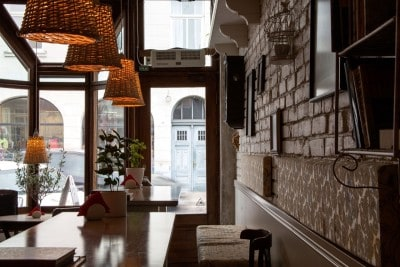You might use area to figure out how many customers a restaurant can comfortably serve.

This leaves an area of 8,000 – (1,600 + 400) = 6,000 square feet for dining space.  Since you want a spacious fine dining experience for your customers, you decide on 20 square feet of space per customer.

This means you will have space for 6,000 / 20 = 300 customers in your restaurant.

### Carpeting

Area is also used to figure out how much carpet to buy to cover a room.  It is also used to figure out the total cost of that carpet.

#### Example: Using Area To Determine Amount Of Carpeting & Cost

Let’s say that you want to carpet 2 bedrooms and a living room in an apartment.  The dimensions of the rooms are as follows:

• Bedroom 1: 12 by 15 feet (area = 12*15 = 180 square feet)
• Bedroom 2: 14 by 13 feet (area = 14*13 = 182 square feet)
• Living Room: 20 by 20 feet (area = 2020 = 400 square feet)

This means that you will need a total area of 180 + 182 + 400 = 762 square feet of carpeting.  Just to be safe you decide to round up and buy 800 square feet of carpet (just in case you need extra if you make a mistake when installing the carpet, or to keep extra on hand if you need to patch a damaged spot later).

At a cost of \$2.50 per square foot, the carpet will cost a total of 800*(\$2.50) = \$2,000.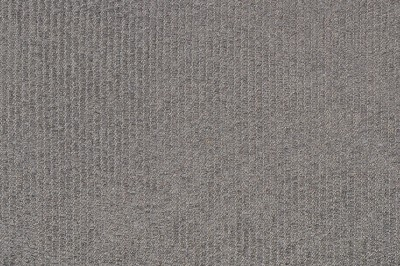You can use area to figure out the amount and cost of carpet you will need for one or more rooms.

### Comparing Rooms, Apartments, & Homes

When you are looking for a room or apartment to rent (or to buy a home), it helps to be able to compare different properties.  That way, you can do a side-by-side comparison of the space you have and the price per square foot.

#### Example: Using Area & Price To Compare Two Homes

Let’s say that you are interested in two homes.  They have the following area (square footage) and prices:

• House A: 1,200 square feet, Price of \$480,000
• House B: 1,500 square feet, Price of \$450,000

We can calculate the price per square foot by division (price / area):

• House A: \$480,000 / 1,200 square feet = \$400 per square foot
• House B: \$450,000 / 1,500 square feet = \$300 per square foot

It looks like House B might be a better deal – not just because it is larger, but because the price per square foot is 75% of House A.

Of course, you might also want to take other factors into account, such as the age, appearance, condition, and location of the house.

### Construction

Area is important in construction, since there are many possible combinations of dimensions to get a room with a given area.

#### Example: Area Of A Room

Let’s say that you want a room with an area of 630 square feet.  There are many possible dimensions, including:

• 15 feet by 42 feet [a very long room – 2.8 times as long as it is wide]
• 18 feet by 35 feet [a room that is about twice as long as it is wide]
• 21 feet by 30 feet [a room that is 70% as wide as it is long]

### Farming & Gardening

Area is important for various calculations in farming and gardening, such as:

• Dimensions of a field or garden
• Amount of fertilizer or lime needed

#### Example: Using Area To Find Out How Much Fertilizer To Use In A Garden

Let’s say that you have a garden that is 30 feet long by 40 feet wide, and you want to add 1.5 pounds of nitrogen per 1000 square feet.

The area of the garden is (30 feet)*(40 feet) = 1200 square feet.

This means we need (1,200 square feet)*(1.5 pounds / 1000 square feet) = 1.8 pounds of nitrogen.

If we are using a fertilizer that is 10% nitrogen by weight (such as 10-10-10), then we would need to add 18 pounds of this fertilizer to the garden, spread evenly over the entire 1,200 foot area (10% of 18 is 1.8, or 1.8 / 0.1 = 18).

### HVAC (Heating & Cooling Buildings)

Area is also useful in calculating the volume of a room or building to figure out how much it will take to heat or cool the space.

For example, if a room has an area of 196 square feet (14 feet by 14 feet) and a ceiling height of 10 feet, then the volume of the room is 196*10 = 1,960 cubic feet.

It will take more energy to heat or cool this room than a room with the same area of 100 square feet but a ceiling height of only 8 feet.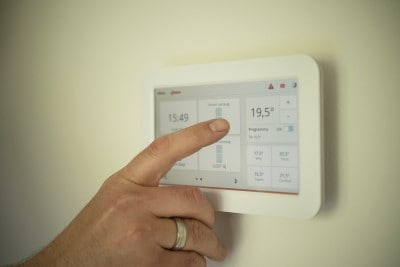Area can help us to figure out how much energy it might cost to heat or cool a room.

### Painting

When painting a house (inside or outside), you will need to know the approximate area of the walls and ceilings that you want to cover.  This will help you to buy enough paint, but not too much.

#### Example: Using Area To Figure Out Amount & Cost Of Paint

Let’s say that you are painting the walls and ceiling of a room with a length of 12 feet, a width of 15 feet, and a height of 8 feet.

Ignoring doors and windows (to give us a little extra paint for touchup or repairs in the future), we need to find the total area we want to paint.

There are 4 walls and the ceiling:

• 2 walls with dimensions 12 feet by 8 feet (length times height), or 2*(12*8) = 192 square feet
• 2 walls with dimensions 15 feet by 8 feet (width times height), or 2*(15*8) = 240 square feet
• Ceiling with dimensions 12 feet by 15 feet (length times width), or 12*15 = 180 square feet

So, the total area you need to cover is 192 + 240 + 180 = 612 square feet.

One gallon of paint covers about 400 square feet, so you will need 612 / 400 = 1.53 gallons.  It makes sense to round up to 2 gallons so that you have extra paint for any future touch-up or repair work.

If a gallon of paint costs \$40, then it will cost you 2*\$40 = \$80 to buy enough paint to cover the 4 walls and ceiling (with plenty left over).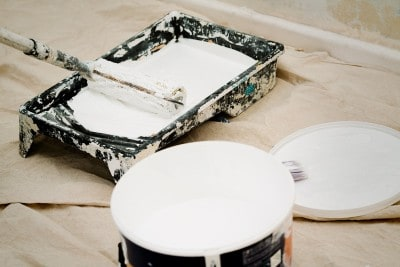Area helps us to figure out the amount and cost of paint for a room (or an entire house).

### Physics

Area is used in several important topics in physics, including:

#### Example: Using Area To Calculate The Pressure On An Object

If you use your hand to press on a wall with a force of 20 Newtons and your hand has an area of 200 square centimeters (0.04 square meters), then the pressure is:

• Pressure = Force / Area = 20 / 0.04 = 500 Newtons per square meter

If you then pushed a piece of metal with an area of 10 square centimeters (0.001 square meters) into the wall with the same force from your hand, then the pressure is:

• Pressure = Force / Area = 20 / 0.001 = 20,000 Newtons per square meter

This means that you can apply 40 times as much pressure on an area that is 1/40th the side of your hand with the same effort.Pushing on a wall with your hand exerts pressure based on the force you use to push and the area of your hand.

### Population Density

The area of a region can also help us to calculate population density (the number of people living in a given area).

#### Example: Using Area To Calculate Population Density

Let’s say that a town has an area of 4 square miles, with 4,000 people living in the town.  This would give a population density of:

• Population Density = Population / Area = 4,000 / 4 = 1,000 people per square mile

### Rainfall

Area can also be used to figure out the volume of rain that will fall in a given area, which might help to predict flood zones.

#### Example: Using Area To Calculate Volume Of Rainfall

In an area that has an area of 1,000 square feet, a storm brings 3 inches of rain over the course of two hours.

The entire volume of rain that falls is the area is:

• Volume = Area*Depth
• Volume = (1,000 square feet)*(3 inches)
• Volume = (1,000 square feet)*(0.25 feet)  [1 foot = 12 inches, so 0.25 feet = 3 inches]
• Volume = 250 cubic feet

So, 250 cubic feet of rain fell in this area over the course of two hours (125 cubic feet per hour).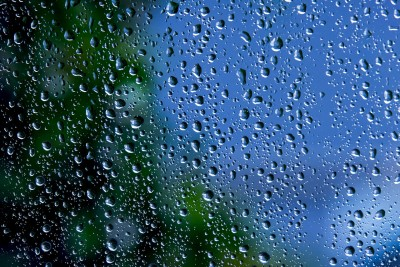We can use area to find the total volume of rain that falls in a given place.

### Solar Panel Power

Area is also useful in figuring out how many solar panels you need to power your house (or how much power you can get from an array with a given area).  Of course, this also depends on sun intensity and the exposure of your panels to the sun.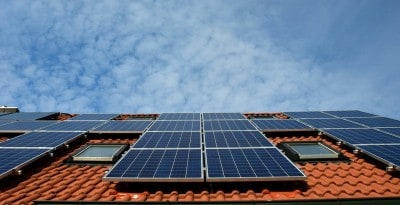Solar panels produce energy based on their area and efficiency, and also based on sun intensity and exposure.

### Valuing Investment Properties & Determining Rent

Area is also useful in figuring out how much rent you might want to charge for a retail space or storage space.  By comparing your space to similar ones on the market, you can get an idea of the value of your space.

#### Example: Using Area To Estimate A Property’s Value

Let’s say that you own a retail space with an area of 10,000 square feet and you want to get an updated estimate of its value (at your banker’s request).

You do some research and find a few comparable retail properties in town.  You inquire and find out that they are renting for \$1.20 to \$1.60 per square foot per month.

This means that your 10,000 square foot space would rent for:

• Low: (10,000 square feet) * (\$1.20 per square foot per month) = \$12,000 per month
• High: (10,000 square feet) * (\$1.60 per square foot per month) = \$16,000 per month

Now you have a range of prices that you might charge for monthly rent (between \$12,000 and \$16,000 per month).

You might charge on the lower end of that range if you want to get the space rented quickly and start earning money.

You might also charge on the higher end if you are willing to wait to find a tenant.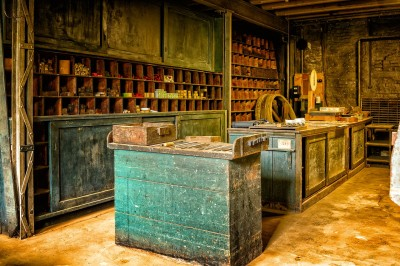Area can help you determine price per square foot and rent for your retail or storage space, based on comparable properties available in the local market.

## Conclusion

Now you know some of the uses of area in real life, along with how to do some of the calculations for those applications.

You can learn about ways to find the area of a square here.

You might also want to check out my article on how circles are used.

You can learn about various uses of algebra in real life here.

I hope you found this article helpful.  If so, please share it with someone who can use the information.

~Jonathon